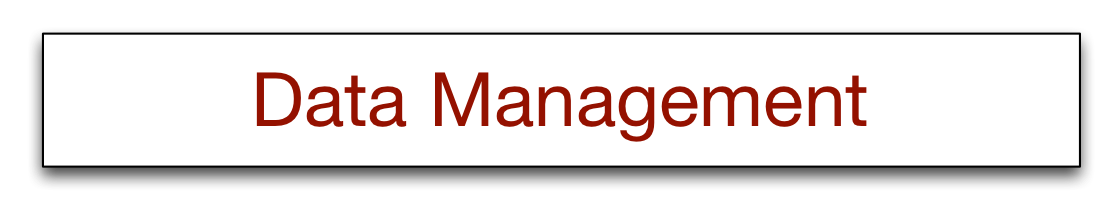# 17 - HDFS and MapReduce Slide Exercises

Use the map component of the mapreduce() to create the cubes of the integers from 1 to 25

```# Conventional solution
# create a list of 25 integers
ints <- 1:25
result <- sapply(ints,function(x) x^3)
result

# MapReduce solution
require(rmr2)
require(reshape)
rmr.options(backend = "local") # local or hadoop
# load a list of 25 integers into HDFS
hdfs.ints = to.dfs(1:25)
# mapper for the key-value pairs to compute squares
mapper <- function(k,v) {
key <- v
value <- c(key^3)
keyval(key,value)
}
# run MapReduce
out = mapreduce(input = hdfs.ints, map = mapper)
# convert to a data frame
df = as.data.frame(from.dfs(out))

#add identifiers for each row as they are consecutively the square and cube
df\$powers <- c('n^3')
output <- cast(df,key ~ powers,value="val")
output```